# Making Sense of It All

In this post, I describe how to use the input from the model created in the previous post. We have a number of detected objects, including their class, location and size. We would like to use this information to track things like scoring a goal, ball possession, ball speed, etc.

The first issue is to get data into Python from the webcam fast enough. Using OpenCV, it is quite easy to read from the webcam:

stream = cv2.VideoCapture(src)


However, this method is very inefficient, because it is running on the main thread, which means that polling the webcam is blocking the rest of the application1. We can move it to a separate thread using features of the imutils package:

stream = WebcamVideoStream(src=0).start()


## Making detections

Now that I have a frame, detecting objects is straightforward using the darknet python library2:

result = darknet.performDetect(frame, makeImageOnly=True, configPath=config, weightPath=weights, metaPath=data)


This gives us an image I’ve shown before: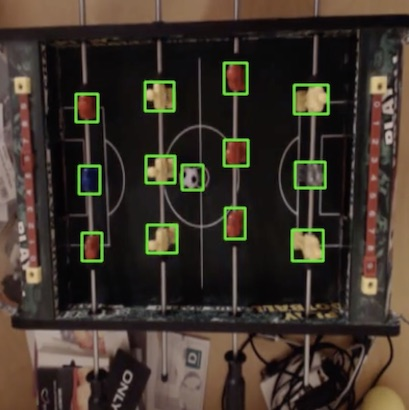The image is not all - we also get a list of detections containing the class, location and size in the format (<class>, <confidence>, (<x>,<y>,<width>,<height>)):

{
'ball': [('ball', 0.9487342834472656,
(129.9288330078125, 168.55563354492188,
21.193037033081055, 31.6497802734375))],
'field_center': [('field_center', 0.9561430811882019,
(205.8816375732422, 180.04901123046875,
18.364641189575195, 25.290817260742188))],
'player': [('player', 0.9998019337654114,
(92.17757415771484, 110.07371520996094,
25.299144744873047, 32.072654724121094)),
('player', 0.9996470808982849,
(165.29103088378906, 122.57108306884766,
15.631088256835938, 35.52423858642578)),
...
]
}


Using this, we can build a representation of the environment which can then be used to calculate statistics, for training the and maybe for visualizing some interesting statistics later.

## Problem: It’s all rotated

There is a potential problem with my setup. Because of the way the camera is installed, we cannot guarantee that the view from above will always show the field consistently with no rotation. When implementing an environment, we would like to be able to assume, e.g. that all players in one row are located exactly beneath each other (i.e. having the same x coordinate), and that the two goals are located directly opposite each other (having coordinates (0, <y>) and (<field length>, <y>), respectively).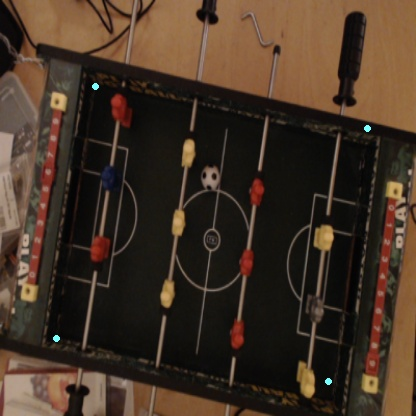Using a few simple calculations, we are able to estimate the location of the corners of the actual board. The following will be used:

• Field center location and size
• One row of players’ locations Using the size of the center, we can calculate the size of the entire table (since we know the physical size of the center and of the entire table). We can estimate the angle of rotation by using a row of players.

Simply put, we calculate the angle between the vector going through the players in the row (the actual rotation) and a vertical vector (the assumed rotation). Using that, we can find the midpoint of the side of the field. Since we know now the angle of rotation and the length of the sides, it is straightforward to estimate the corners’ location.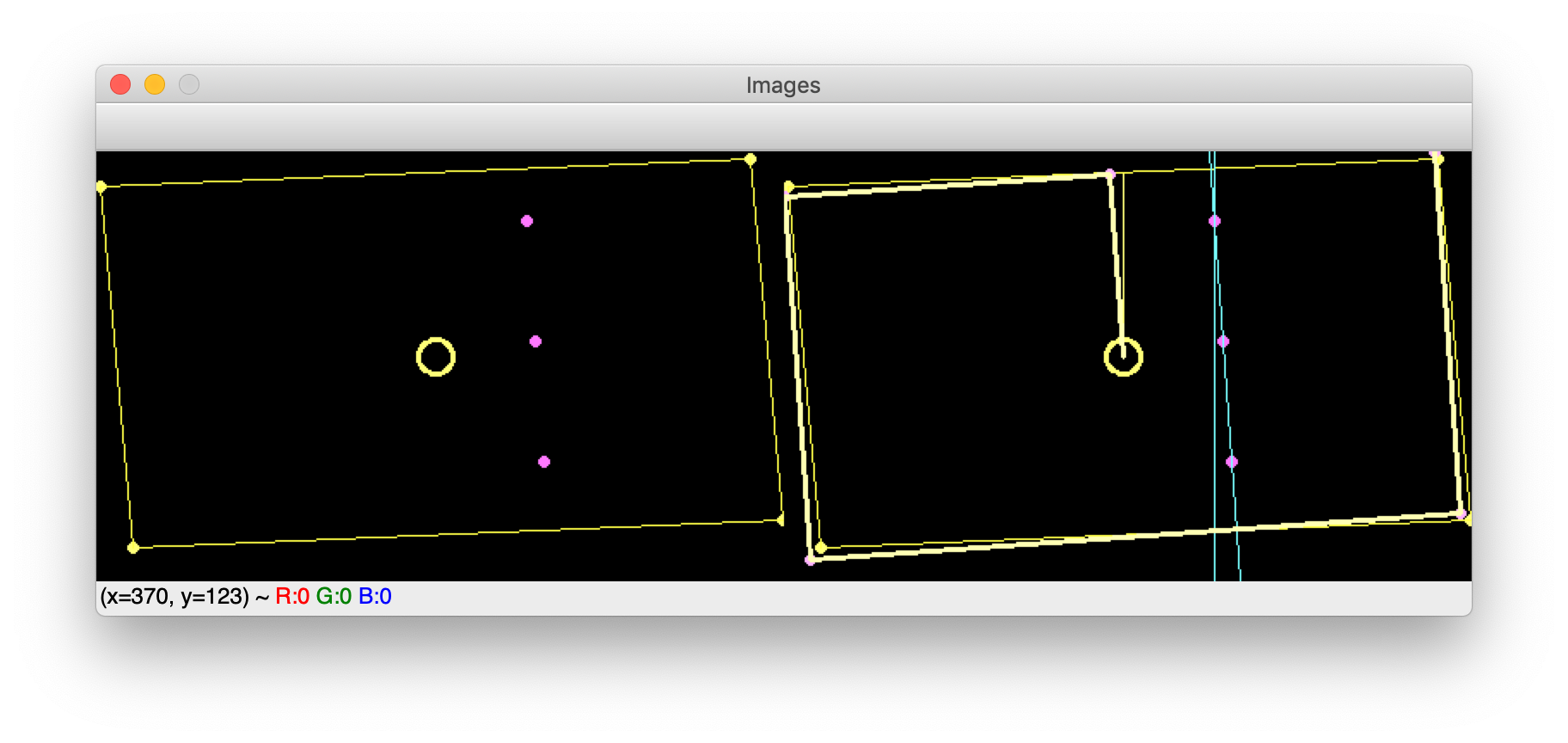The figure shows on the left side a representation of a rotated table with a row of players. On the right side, we show the calculated vectors and how they estimate the corner location of the actual table.

## Transforming positions

Using the coordinates of the corners, all that is left to do is to transform the detected locations into the new coordinate system. However, I have chosen a bit different method. I do a four-point perspective transformation3, which takes as input the image and the four calculated corners. As an output, we get a transformed image that only contains the field (within the corners) and is warped into a rectangular shape. We thus obtain a consistent image containing only the field. To obtain the location of ball and players, we use Yolo once again and voila: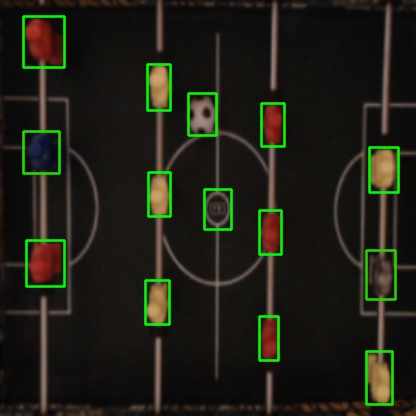My reason for doing like this is:

• We only detect the corners once (assuming that the camera does not move during a match)
• The rest of the time, we use OpenCV to get a consistent picture of the field, ensuring that no erroneous detections will be made outside the field (e.g. a hand being confused with a player)

There may be many other ways to solve this, but it was a fun little exercise and the result is satisfactory.

## The final environment

Using this method, we now have a consistent representation of the field and an environment which at all times contains updated information about ball and player location: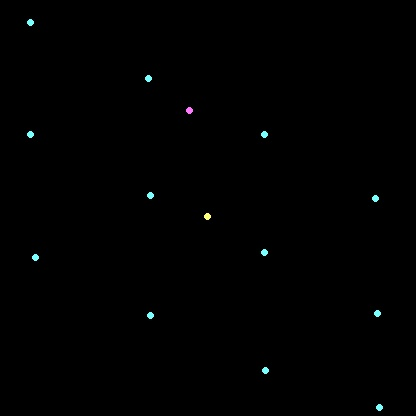Using Flask, I created a small web-application to show the stream and send commands to the system. I created a route for publishing the feed using Motion JPEG4, which will take the latest image produced from the system (e.g. the environment or the raw detections) and add it to the stream. Further, to allow recalculation of the corners on demand, it is possible to add a route to do so.

def gen(image):
while True:
frame = d.snapshots.get(image)
if frame is not None:
_, jpeg = cv2.imencode('.jpg', frame)
yield (b'--frame\r\n'
b'Content-Type: image/jpeg\r\n\r\n' + jpeg.tobytes() + b'\r\n\r\n')

@app.route('/video/<feed>')
def video(feed):
return Response(gen(feed),
mimetype='multipart/x-mixed-replace; boundary=frame')

@app.route('/recalculate')
def recalculate():
d.schedule_recalculation()

return "ok"


## Next steps

With a consistent environment, the next step will be to calculate a few simple statistics like ball possession, goals and ball speed and present those in the web-application.

The next step after that is to start building the actual robot! I am very eager to begin, but a start, I will probably add an interface in the web-application to control the robot, just to verify that everything works. After that, the real fun begins: build an autonomous controller that can actually play (and hopefully well!).

### Footnotes

1. More details available here

2. I have made a few adjustments, so that the script is not required to be run directly in the darknet folder.

• Set DARKNET_DIR to the folder where Darknet is compiled.
• Set YOLO_DIR to the folder containing the Yolo-model for Table-soccer.